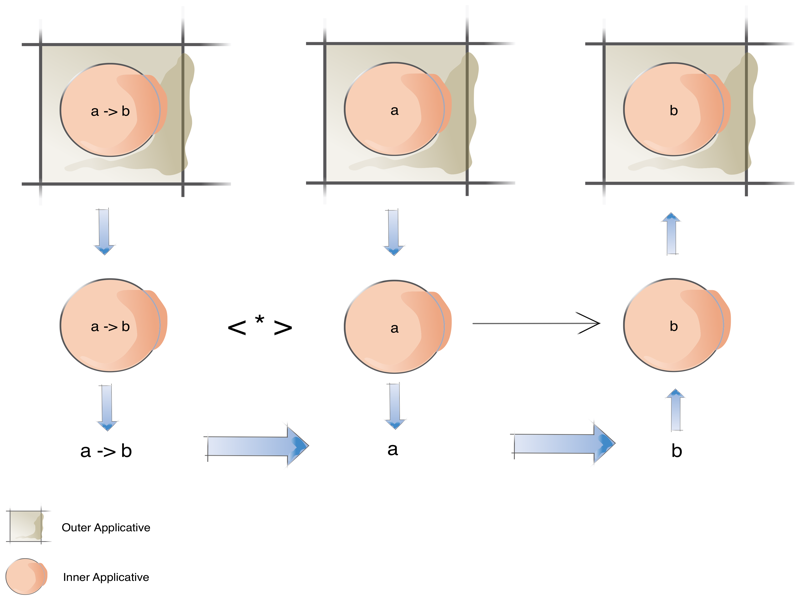While reading The Gentle Introduction to Monad Transformers I came across an interesting use of liftA2. To refresh our memories, liftA2 is defined as:

``liftA2 :: Applicative f => (a -> b -> c) -> f a -> f b -> f c``

It basically lifts a binary function, let’s call it g, across two Applicative Functors (f a and f b) that each have one of the parameters g requires (a and b respectively). It then produces the result of applying g(a b) in a third Applicative Functor (f c).

So a simple example would be something like:

``liftA2 (+) (Just 5) (Just 6) = Just 11``

All very easy so far.

What does liftA2 (<*>) do?

Before we answer that question, let’s explore the <*> operator.

The starship operator (as I like to call it) from Applicative is defined like this:

``<*> :: Applicative f => f (a -> b) -> f a -> f b``

The starship operator takes a unary function, let’s call it h, that’s within a Applicative Functor (f ( a -> b )) and applies it to a value (a) also in an Applicative Functor (f a). It then returns the result of function application (h a) in another Applicative Functor (f b).

A simple example of its use would be something like:

``(Just (+5)) <*> (Just 6) = Just 11``

Again very simple.

So liftA2 lifts a binary function into two Applicative Functors to get its result. The starship operator applies a function that requires one value within an Applicative Functor into another Applicative context that has the value it needs.

So back to our question: What does liftA2 (<*>) do?

We can see from the above that liftA2 works on Applicative Functors and the starship operator also works on Applicative Functors. It might be useful when we have nested Applicative Functors.

Wait … what?

Continuing with our example, say we had this:

``````let v1 = IO (Just (+5))
let v2 = IO (Just 6)``````

How could we apply the nested +5 function to the nested 6 value to retrieve our result of 11?

With the power of listA2 boosted with starship power we could do:

``liftA2 (<*>) v1 v2 = IO (Just 11)``

That seemed really easy. :)

And now for a boxes and circles diagram:diagram of how liftA2 works with the starship operator

Using liftA2 (<*>) we can simply apply functions within nested Applicatives to values also within nested Applicatives.